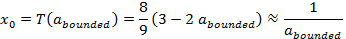Newton-Raphson Approximation

INTRODUCTION

Perhaps the best known method for finding the roots of a function is the Newton-Raphson approximation.   Its popularity stems from a relative simplicity, a fast quadratic convergence, and an ability to handle not just polynomials but any non-linear function for which we can calculate or approximate a derivative.

There are technically better algorithms but they tend to be complex and not as easily implemented.  The only minor disadvantage is having to find an initial approximation sufficiently near the final result.

HISTORY

The method was anticipated in 1600 by Francois Vieta (1540–1603) who had published a simple perturbation technique for the solution of polynomial equations.  The first publication of Newton's much improved work describing an algebraic method to find polynomial roots was published by John Wallis in 1685 as “A Treatise of Algebra both Historical and Practical.”   In 1690, Joseph Raphson published a simplified version to include successive approximations entitled “Analysis Aequationum Universalis.”   Finally, in 1740, Thomas Simpson described Newton's method in its modern form as an iterative method for solving general nonlinear equations involving differential calculus.

ALGORITHM

We first write an equation f(x) whose solution would give us the desired functionality.   The algorithm then generates a numerical approximation of the roots, meaning values of the independent variable x for which the function is zero.

We start with an initial guess “x0” and then repeatedly apply a recursion relation.  That is, for any given approximation “xi” we calculate a value “xi+1” that is closer to the exact answer.  And the convergence is quadratic which means the number of correct digits doubles for each iteration.   Eventually we get a value “xn” which is close enough to the root so that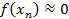The recursion equation is derived from a simple result of differential calculus in which the derivative of this function at the approximate value “xi“ can be written asSince the derivative is the slope of the tangent line to the function at “xi“,  we can extend this tangent line to intercept the x-axis, so from our last guess of “xi” we get a better approximation “xi+1”.  This is graphically displayed below.   Note that this assumes the derivatives of the function are sufficiently “smooth” between the initial guess “x0” and the final result “xn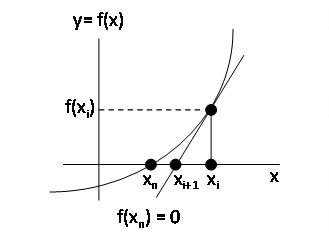And the recursion equation is unchanged even if the slope is negative instead of positive.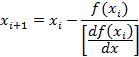We repeat this process multiple times so starting from an initial guess, x0, we getAfter a few such iterations, we are for all practical purposes close enough and so we declare victory and go home.

SQUARE ROOT

If we want to numerically calculate the square root of some parameter “a” for a > 0, we can express this functionality in the equation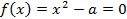The value of “x” which makes f(x) equal to zero is the square root of “a”.   The derivative of this function is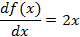And the next best approximation is given by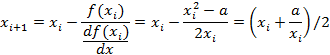At this point we want to restrict the allowed values of the parameter “a” to lie within the range from 1 to 4.  This is because we have only a limited number of digits and the smallest range will give us the greatest and most consistent accuracy.   Basically we want to eliminate leading and trailing zeros and deal only with significant, or non zero, digits.

We choose this interval because any value of “a” can be expressed as a multiple of two terms, that is some number between 1 and 4 times a second term which is an integral power of 4.  This can be written as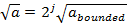On a computer for numbers expressed in binary notation, a division or multiplication by two or four or by any power of two is easily accomplished by simply shifting the decimal point.

The only remaining issue is then to find a starting value “x0” for any give value of the parameter “a”.  Since this is a somewhat different exercise with much less precision than the method described above we will use a different independent variable “z”.

One generally acceptable method is to approximate the square root of z with a straight line, or linear, curve fit for values of z from 1 to 4.  We further assume that the best approximation is that which minimizes the total squared error across the entire range.  So we will ignore other techniques that might instead minimize, or bound, the maximum error.   The function of interest is then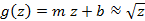where “m” is the slope of the straight line and “b” is the y-axis intercept.   The total squared error is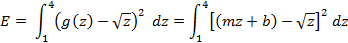Or after some work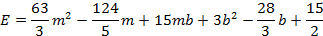And to find the minimum of E with respect to “m” and “b” we set the partial derivatives so zero as follows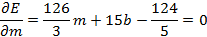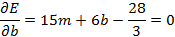The solution of these two simultaneous equations is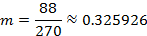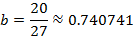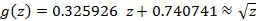So we can summarize the algorithm in the following steps

1.      Return with an error if a<0

2.      Return a value of zero if a=0

3.      Factor the parameter “a” where “j” is some arbitrary integer

“abounded”  = a/4j  where4.      Get a guess of the initial value, “x0” using our straight line curve fit5.      Repeated apply the iteration to get a much more precise value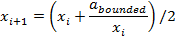6.      Stop when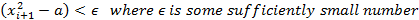Or we could also stop when we observe that the new value of xi+1 doesn’t change much.

7.      Multiply the final value of “xn” by 2j so that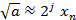DIVISION

To calculate the inverse of some parameter “a” we can write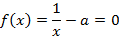And the derivative and recursion relation is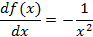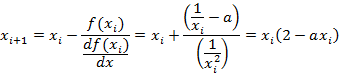Since we work with a limited number of digits, for greatest accuracy, we will shift the value of ”a” to lie within the interval of from ½ to 1.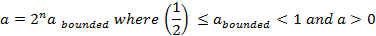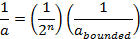But again for binary numbers in a computer, division by two is simply a shift of the decimal point.

To get an initial estimate, we might fit a straight line as before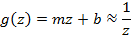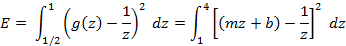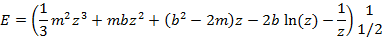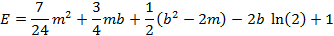And as before we use set the partial derivatives to zero to find the minimum value of E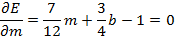And the solution is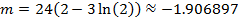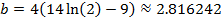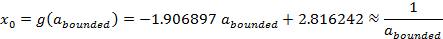And the algorithm is similar to that given before except that we shift the input argument to the range from ½ to 1 for greatest accuracy with a limited number of digits.

Note that a simpler, if less accurate, approximation for the initial guess of “x0” can be obtained using Taylor’s Series.  In general, any function T(z) can be expanded about “h” as follows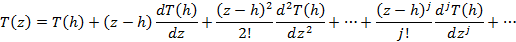For the specific function where T(z) is simply the inverse of x, we haveSince our range of z is from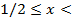1, we will set h = 3/4 so as to be in the middle of the range.  And then keeping just the first two terms we have